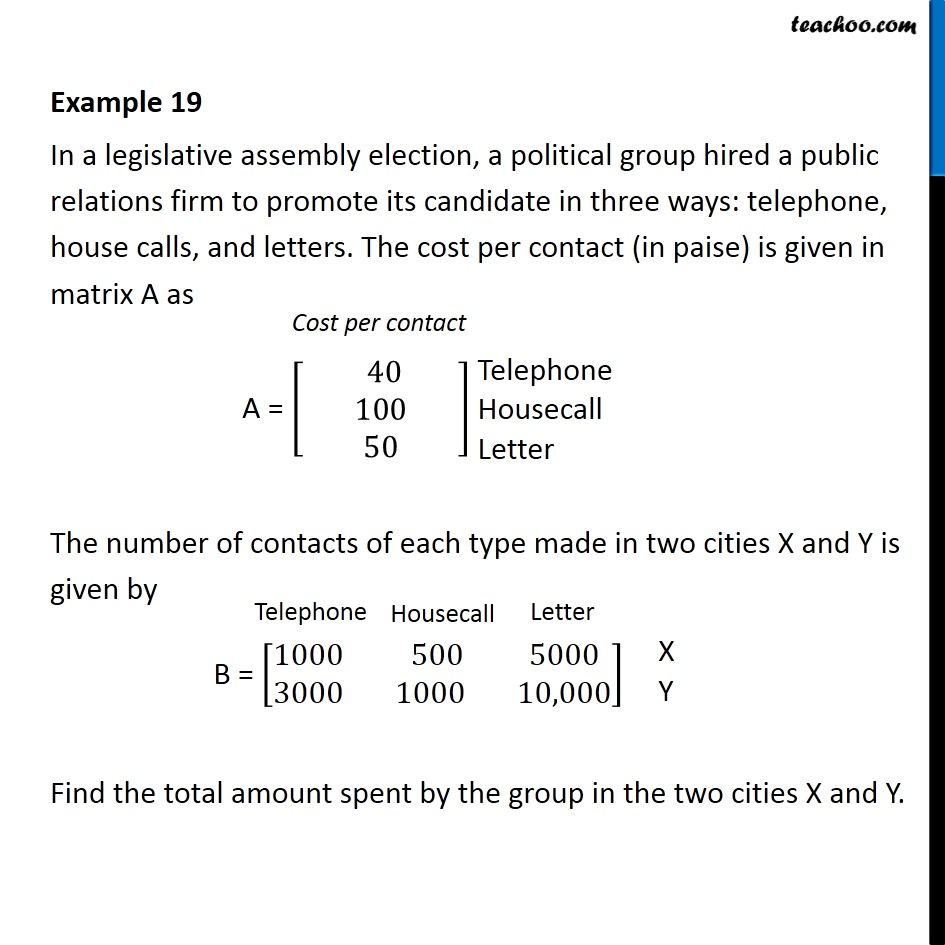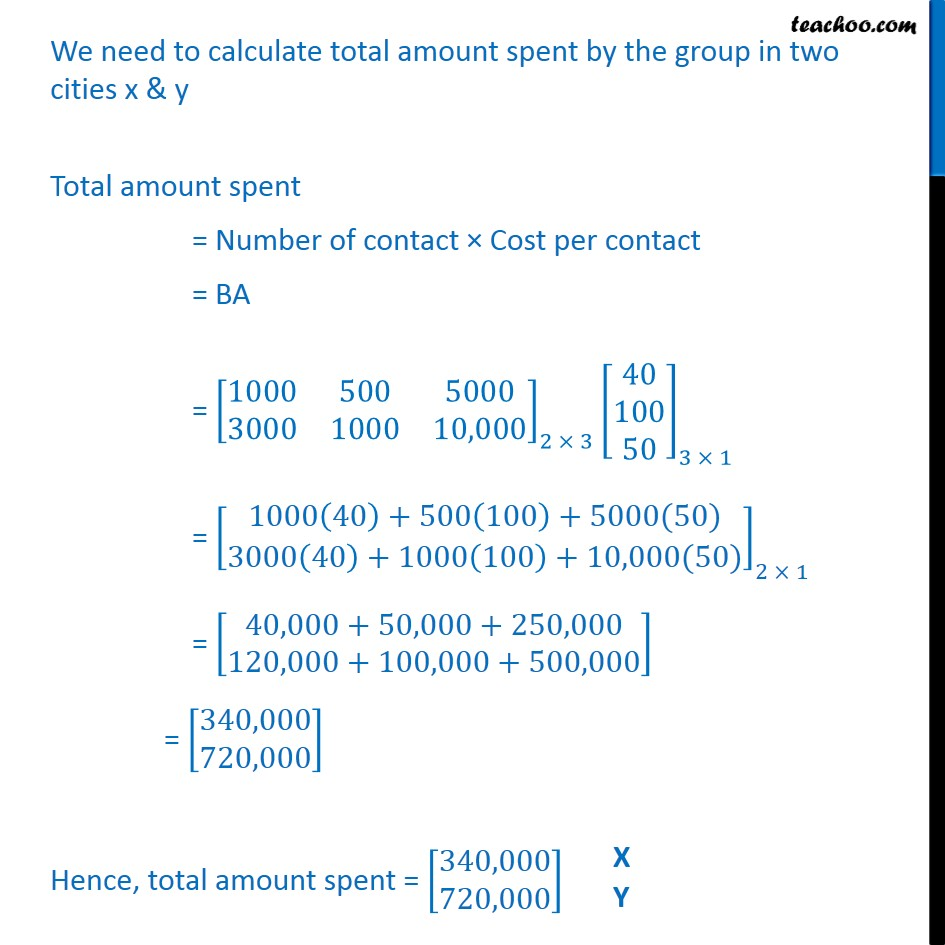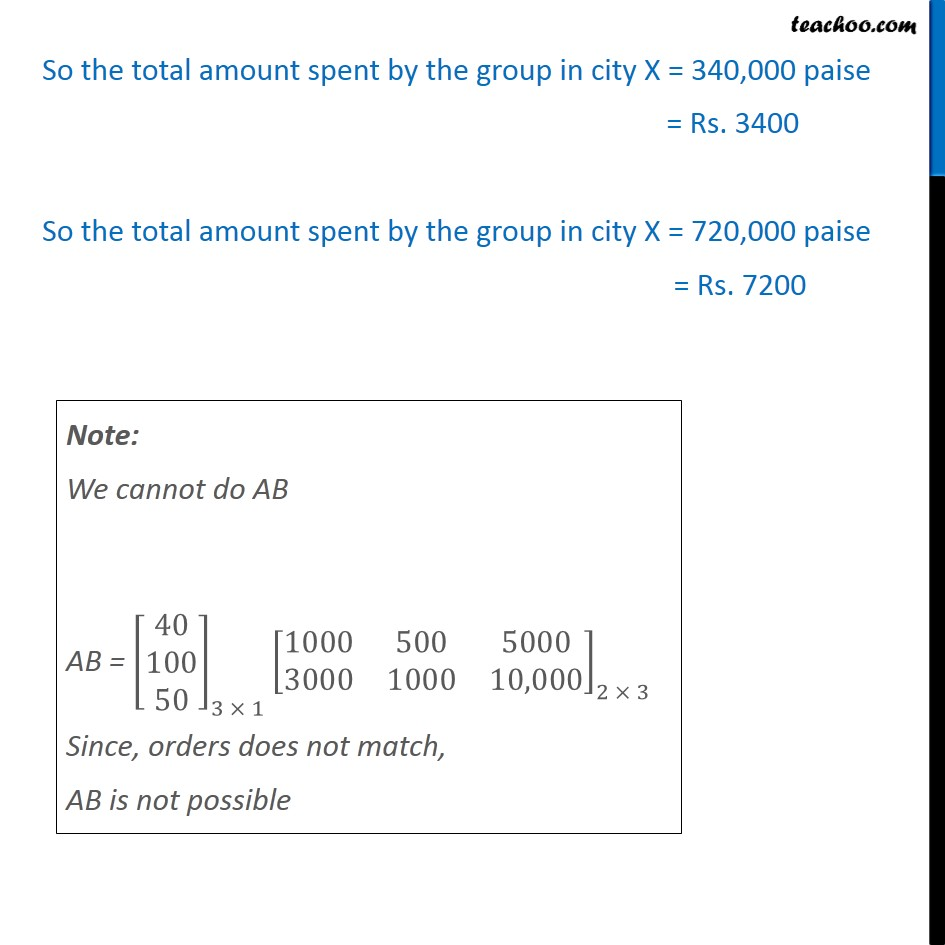Examples

Chapter 3 Class 12 Matrices (Term 1)
Serial order wiseNow learn Economics at Teachoo for Class 12

### Transcript

Example 19 In a legislative assembly election, a political group hired a public relations firm to promote its candidate in three ways: telephone, house calls, and letters. The cost per contact (in paise) is given in matrix A as A = [■8( 40 @100@50)] The number of contacts of each type made in two cities X and Y is given by B = [■8(1000& 500 &5000@3000&1000&10,000)] Find the total amount spent by the group in the two cities X and Y. We need to calculate total amount spent by the group in two cities x & y Total amount spent = Number of contact × Cost per contact = BA = [■8(1000&500&5000@3000&1000&10,000)]_(2 × 3) [■8(40@100@50)]_(3 × 1) = [█(1000(40)+500(100)+5000(50)@3000(40)+1000(100)+10,000(50))]_(2 × 1) = [█(40,000+50,000+250,000@120,000+100,000+500,000)] = [█(340,000@720,000)] Hence, total amount spent = [█(340,000@720,000)] So the total amount spent by the group in city X = 340,000 paise = Rs. 3400 So the total amount spent by the group in city X = 720,000 paise = Rs. 7200